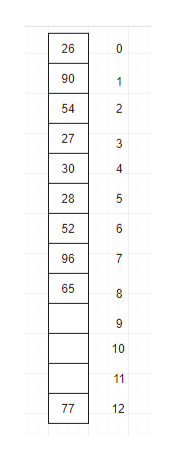# Exercise 1Consider a hash table of size 13, and assume open addressing with linear probing Show what the hash table will look like after the following items are added in the order shown.26 54 77 90 27 30 28 52 96 65  What’s the load factor of the hash table after the values above are added?   If any of the values cause a collision, indicate which.   How many comparisons are needed to find 65 in the table? Briefly explain.   How many comparisons are needed to determine that 104 is not in the table? Briefly explain.   What’s the hash value computed for the string “dog”? Show your work.    Exercise 2Suppose chaining is used as the method for collision resolution.Part AShow what the hash table will look like after the following items are added in the order shown.26 54 77 90 27 30 28 52 96 65       How many comparisons are needed to find 65 in the table?    How many comparisons are needed to determine that 104 is not in the table? Briefly explain.    Exercise 3Consider a hash table with a load factor of 0.8. What’s the average number of comparisons for a successful search assuming open addressing with linearprobing?  What’s the average number of comparisons for a unsuccessful searchgiven the same assumptions?

Question
168 views

Exercise 1

Consider a hash table of size 13, and assume open addressing with linear probing

1. Show what the hash table will look like after the following items are added in the order shown.

26 54 77 90 27 30 28 52 96 65

1. What’s the load factor of the hash table after the values above are added?

1. If any of the values cause a collision, indicate which.

1. How many comparisons are needed to find 65 in the table? Briefly explain.

1. How many comparisons are needed to determine that 104 is not in the table? Briefly explain.

1. What’s the hash value computed for the string “dog”? Show your work.

Exercise 2

Suppose chaining is used as the method for collision resolution.

Part A

1. Show what the hash table will look like after the following items are added in the order shown.

26 54 77 90 27 30 28 52 96 65

1. How many comparisons are needed to find 65 in the table?

1. How many comparisons are needed to determine that 104 is not in the table? Briefly explain.

Exercise 3

1. Consider a hash table with a load factor of 0.8. What’s the average number of comparisons for a successful search assuming open addressing with linear

probing?

1. What’s the average number of comparisons for a unsuccessful search

given the same assumptions?

check_circle

Step 1
1.
• As the given size of the table is 13, as well as the number of elements to be inserted in the table is 10 (26, 54, 77, 90, 27, 30, 28, 52, 96, 65).
• Insert element in hash table by using formula <element> MOD <size of hash table> (it returns remainder as a result).
• And on the basis of the result from the above formula for every element, the element will be stored accordingly.help_outlineImage Transcriptionclose26 0 90 1 54 2 30 4 28 5 52 6 96 7 65 8 9 10 11 77 12 N LO CO 27 fullscreen
Step 2

2.

• The load factor of the above table will be calculated, which tells the number of collisions probability.
• The load factor of a specific hash table directly proportional to element collision in the hash table. And the formula is:

### Want to see the full answer?

See Solution

#### Want to see this answer and more?

Solutions are written by subject experts who are available 24/7. Questions are typically answered within 1 hour.*

See Solution
*Response times may vary by subject and question.
Tagged in

### Coding Theory